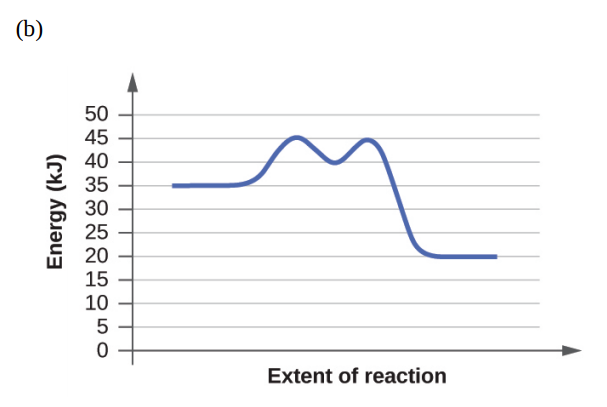# Problem: For each of the following reaction diagrams, estimate the activation energy (Ea ) of the reaction:

⚠️Our tutors found the solution shown to be helpful for the problem you're searching for. We don't have the exact solution yet.

###### Problem Details

For each of the following reaction diagrams, estimate the activation energy (Ea ) of the reaction: